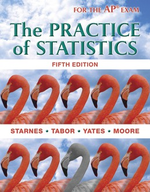### Solution Found!# Olympic figure skating For many people, the womens figure skating competition is the

Chapter 3, Problem 55

(choose chapter or problem)

Get Unlimited Answers! Check out our subscriptions
QUESTION:

For many people, the women’s figure skating competition is the highlight of the Olympic Winter Games. Scores in the short program x and scores in the free skate y were recorded for each of the 24 skaters who competed in both rounds during the 2010 Winter Olympics in Vancouver, Canada. A regression analysis was performed using these data. The scatterplot and residual plot follow. The equation of the least-squares regression line is $$\hat{y}=-16.2+2.07 x$$. Also, $$s=10.2$$ and $$r^{2}=0.736$$(a) Calculate and interpret the residual for the gold medal winner, Yu-Na Kim, who scored 78.50 in the short program and 150.06 in the free skate.

(b) Is a linear model appropriate for these data? Explain.

(c) Interpret the value of s.

(d) Interpret the value of $$r^2$$

QUESTION:

For many people, the women’s figure skating competition is the highlight of the Olympic Winter Games. Scores in the short program x and scores in the free skate y were recorded for each of the 24 skaters who competed in both rounds during the 2010 Winter Olympics in Vancouver, Canada. A regression analysis was performed using these data. The scatterplot and residual plot follow. The equation of the least-squares regression line is $$\hat{y}=-16.2+2.07 x$$. Also, $$s=10.2$$ and $$r^{2}=0.736$$(a) Calculate and interpret the residual for the gold medal winner, Yu-Na Kim, who scored 78.50 in the short program and 150.06 in the free skate.

(b) Is a linear model appropriate for these data? Explain.

(c) Interpret the value of s.

(d) Interpret the value of $$r^2$$

Step 1 of 6

Given,

Number of skaters, $$n=24$$

The standard deviation of the residuals, $$s=10.2$$

The coefficient of determination, $$r^{2}=0.736$$

The equation of the least-squares regression line for predicting scores in the free skate from the scores in the short program,

$$\hat{y}=-16.2+2.07 x$$

Using the scatterplot and residual plot we have to determine the following: